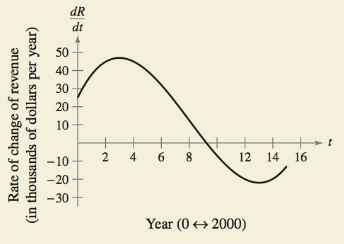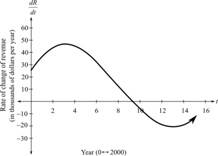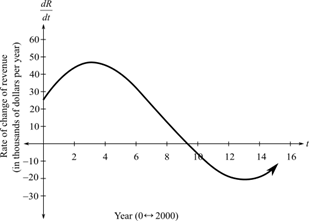Chapter 5.2, Problem 58E### Calculus: An Applied Approach (Min...

10th Edition
Ron Larson
ISBN: 9781305860919

#### Solutions

Chapter
Section### Calculus: An Applied Approach (Min...

10th Edition
Ron Larson
ISBN: 9781305860919
Textbook Problem
14 views

# HOW DO YOU SEE IT? The graph shows the rate of change of the revenue of a company from 2000 through 2015.(a) Approximate the rate of change of the revenue in 2004. Explain your reasoning.(b) Is R(7) − R(6) > 0? Explain your reasoning.(c) Approximate the years in which the graph of the revenue is concave upward and the years in which it is concave downward. Approximate the years of any points of inflection.

(a)

To determine

To calculate: The approximate rate of change of the revenue in the year 2004 from the provided graph.Explanation

Given Information:

The provided graph is,

Calculation:

Consider the provided graph.

In order to find the value of the rate of change of revenue dRdt in the year 2004 that is at t=4,

Locate the point t=4 on the horizontal axis and move

(b)

To determine

Whether the value of R(6)R(7) is greater than 0 or not from the provided

graph.(c)

To determine

To calculate: The years in which the graph of the revenue is concave upward, concave

downward and the years of any point of inflection.

### Still sussing out bartleby?

Check out a sample textbook solution.

See a sample solution

#### The Solution to Your Study Problems

Bartleby provides explanations to thousands of textbook problems written by our experts, many with advanced degrees!

Get Started

#### Evaluate the limit, if it exists. limh0(x+h)x3h

Single Variable Calculus: Early Transcendentals, Volume I

#### Simplify the expressions in Exercises 97106. x1/2yx2y3/2

Finite Mathematics and Applied Calculus (MindTap Course List)

#### converges to: 2 the series diverges

Study Guide for Stewart's Multivariable Calculus, 8th

#### Simplify. 10c3(8c2d+12)

Mathematics For Machine Technology